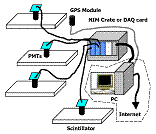## Research Papers in Physics and Astronomy## Gregory Snow Publications

2-4-2014

#### Citation

JHEP02(2014)013; doi:10.1007/JHEP02(2014)013

#### Comments

Copyright © 2014 CERN, issued under cc license; published by Springer.

#### Abstract

Measurements are presented of the associated production of a W boson and a charm-quark jet (W + c) in pp collisions at a center-of-mass energy of 7TeV. The analysis is conducted with a data sample corresponding to a total integrated luminosity of 5 fb−1, collected by the CMS detector at the LHC. W boson candidates are identified by their decay into a charged lepton (muon or electron) and a neutrino. The W + c measurements are performed for charm-quark jets in the kinematic region pjetT > 25 GeV, |ɳjet| < 2.5, for two different thresholds for the transverse momentum of the lepton from the W-boson decay, and in the pseudorapidity range |ɳ| < 2.1. Hadronic and inclusive semileptonic decays of charm hadrons are used to measure the following total cross sections: σ(pp → W+ c + X) × B(W → ν) = 107.7 ± 3.3 (stat.) ± 6.9 (syst.) pb (pT > 25 GeV) and σ (pp→ W+ c + X)×B(W→ν) = 84.1 ± 2.0 (stat.) ± 4.9 (syst.) pb (pT > 35 GeV), and the cross section ratios σ (pp → W+ +¯c + X)/ σ (pp → W + c + X) = 0.954 ± 0.025 (stat.) ± 0.004 (syst.) (pT > 25 GeV) and σ (pp → W+ + c + X)/ σ (pp → W + c + X) = 0.938 ± 0.019 (stat.) ± 0.006 (syst.) (pT > 35 GeV). Cross sections and cross section ratios are also measured differentially with respect to the absolute value of the pseudorapidity of the lepton from the W-boson decay. These are the first measurements from the LHC directly sensitive to the strange quark and antiquark content of the proton. Results are compared with theoretical predictions and are consistent with the predictions based on global fits of parton distribution functions.

COinS